Next: Introduction

# Towards the Interpretability Logic of all Reasonable Arithmetical Theories

Joost J. Joosten

12 december 1998

### Abstract:

The subject of this paper is the interpretability logic of all reasonable arithmetical theories, which we baptize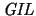. This logic has long been conjectured by Albert Visser to be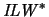. No modal completeness result was known for. In order to provide a completeness proof of, a good understanding of the sublogic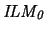seems indispensable. But there was no modal completeness result foreither. In this paper a method for constructing models is developed. By this model-construction method we obtain a new proof of the modal completeness and decidability of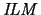. Moreover do we obtain the modal completeness of. Furthermore, a new principle, P0, is introduced and studied. This principle is seen to be arithmetically valid and is completely independent with regard to the other principles studied here. The new logic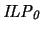turns out to be modally incomplete. The conjecture ofbeing the interpretability logic of all reasonable arithmetical theories is thus falsified.

Joost Joosten
2000-02-07